x

## How to Create (randomized) Math Questions - Quiz Builder Tutorial

Posted on Wednesday, August 24th 2022*Introduction

So you’ve created a quiz, and you want to use Arlinear’s math suite in order to build math questions for your students.

In this article, we will cover building different math questions and using randomized variables within your questions

*Create a Math Question

Start by creating a math question by hovering over “+ Add Question” and clicking “Math”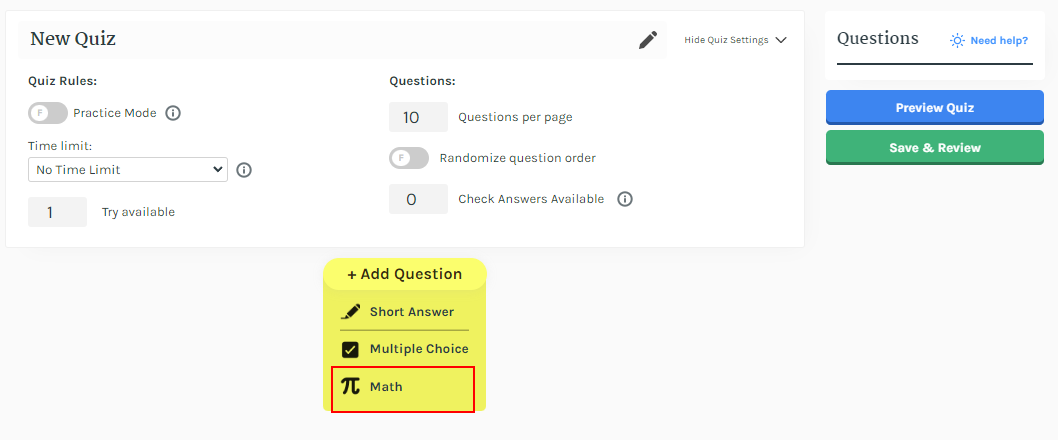*Compute Operations

Compute Operations require the student to calculate the answer to the defined math question.

1. Click on “+ Compute Operation” and type 1+1 in the box.

Pro Tip: When interpreting math, Arlinear accepts a wide variety of inputs. Try typing “1 plus 1” or even “one plus one” .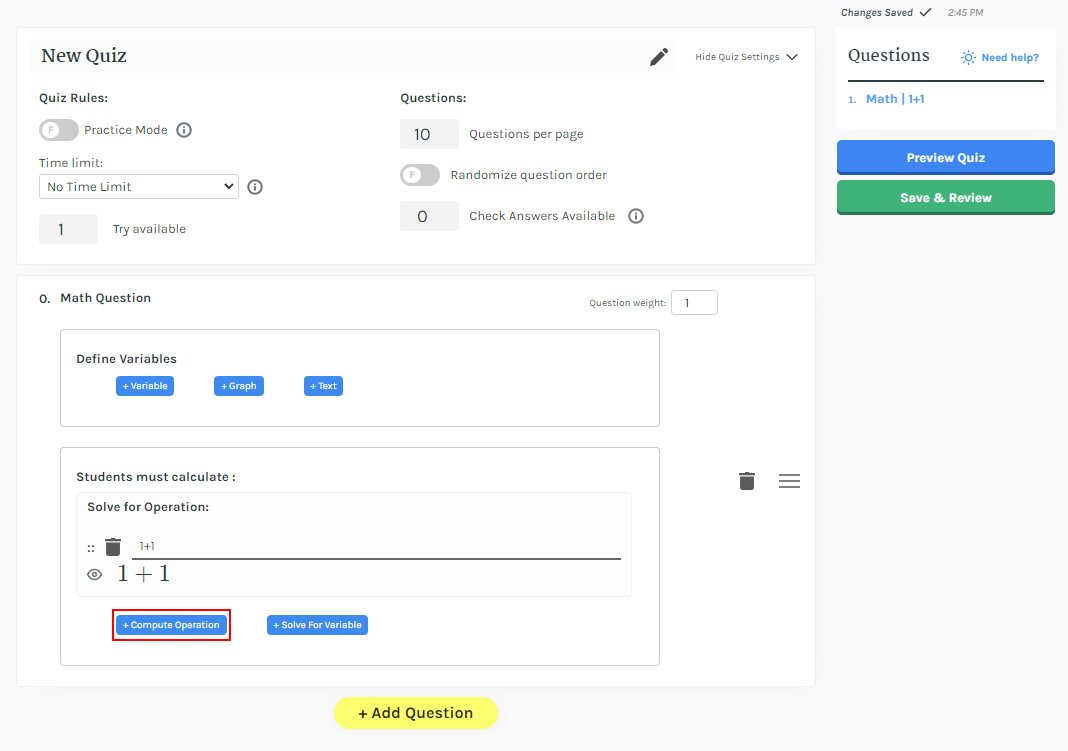*Solve for X

The “Solve for x” problem type is very common for a variety of subjects. Let's start with a very simple example.

1. Click “+Solve For Variable”.

1. Type “x^2+5x+6” into the left side of the equality, and “0” into the right side.

Hint: The “^” symbol means “to the power of”. So typing “x^2” will give the same results as typing “x squared”

We want students to find all values of x, so set the value of the drop-down to “equal to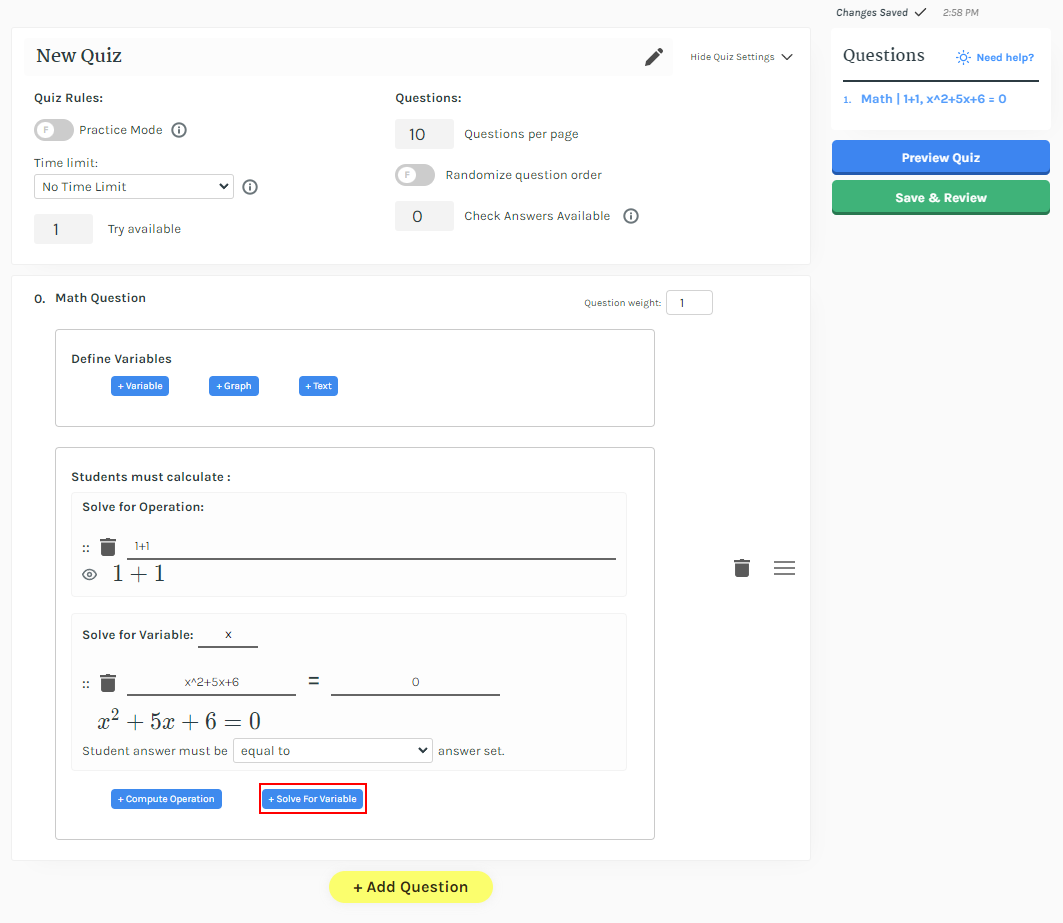*Variables

Defining variables in the quiz builder works the same as in mathematics.

1. Create a variable a by clicking “+ Variable”, then set its value to 2. The variable can be placed in any following math entry.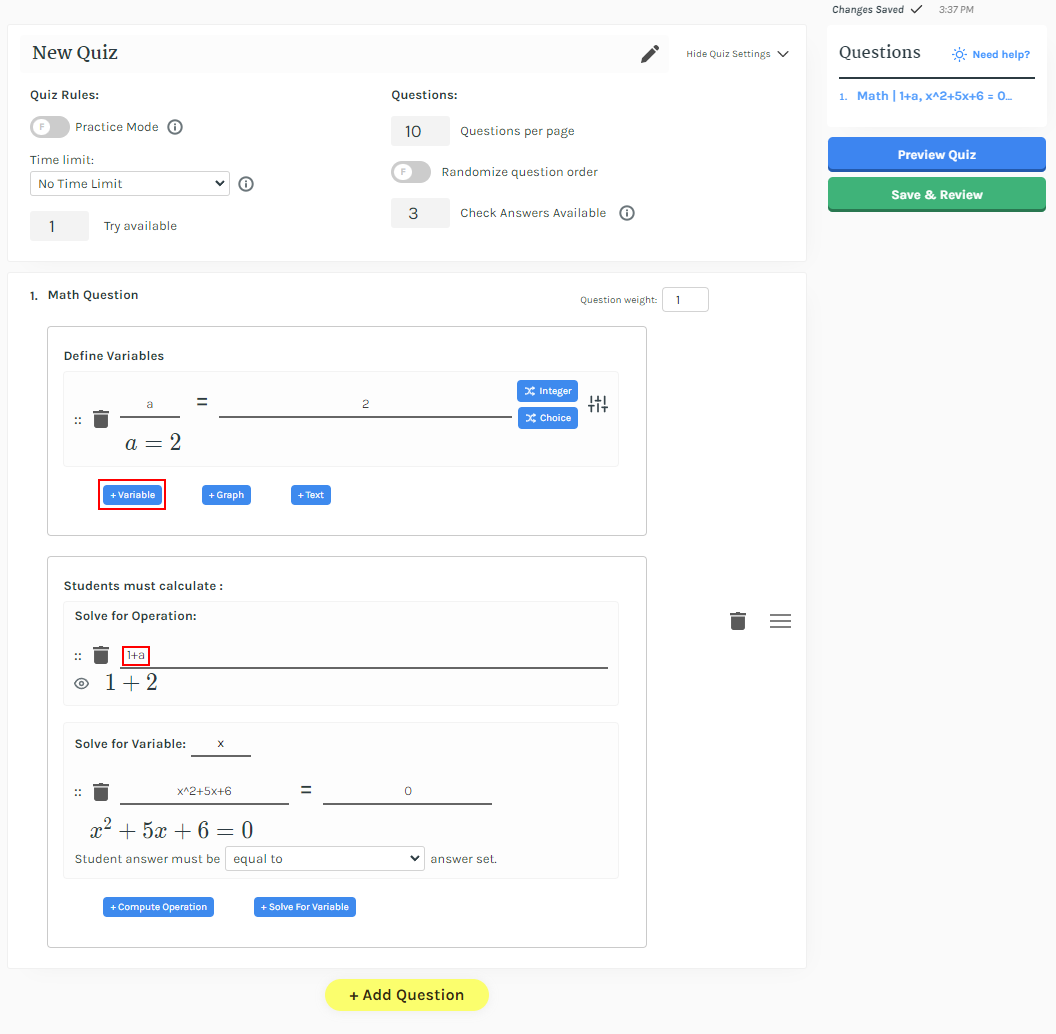1. Click the “Random Integer” button to make your variable a random integer. For each student, your variable will take on a different value. Let's make   random variable between 1 and 5.

Pro tip: "RandomInteger[{1,5}]" gives a number between 1 and 5, try changing the 1 and 5 for different results.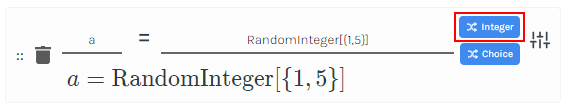1. Set the value of “Check Answers Available” to 3, and click “Preview Quiz”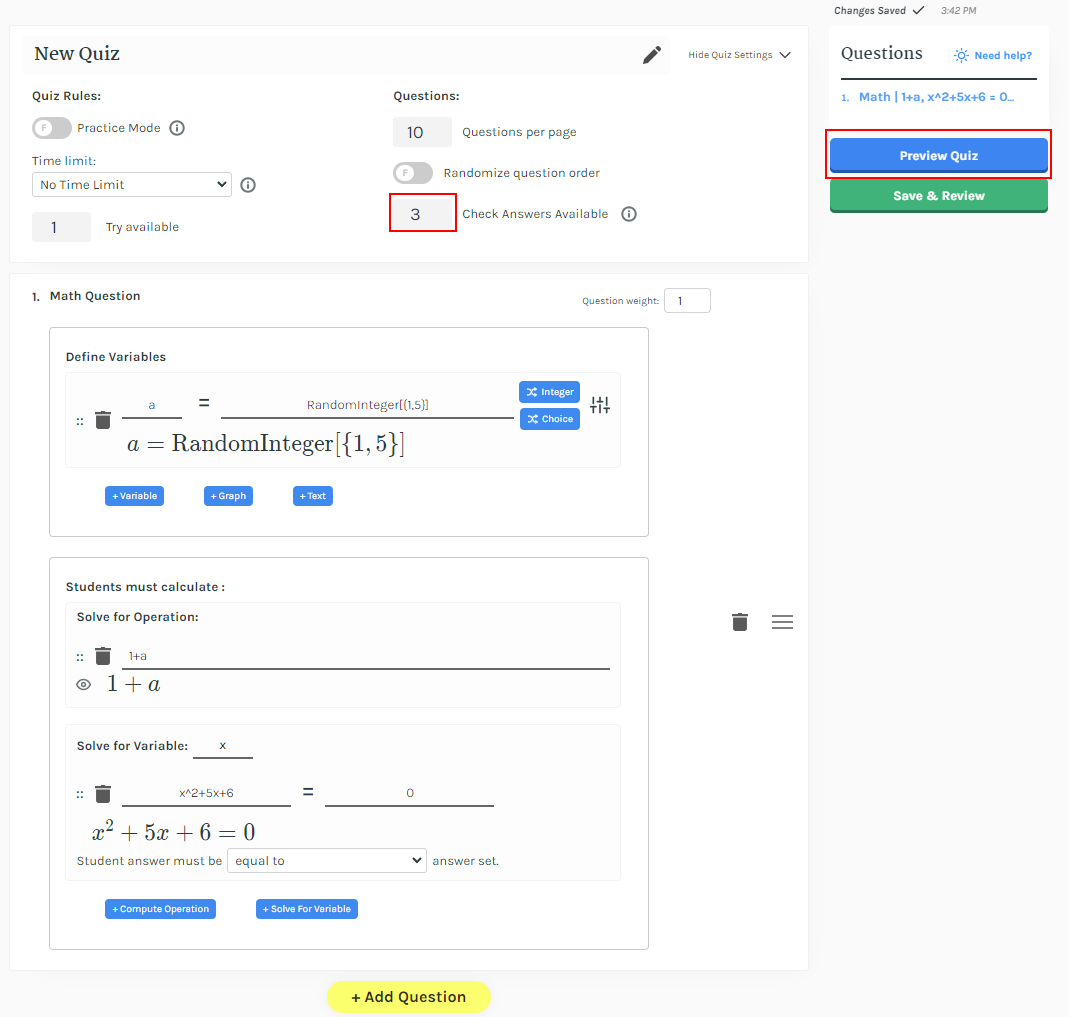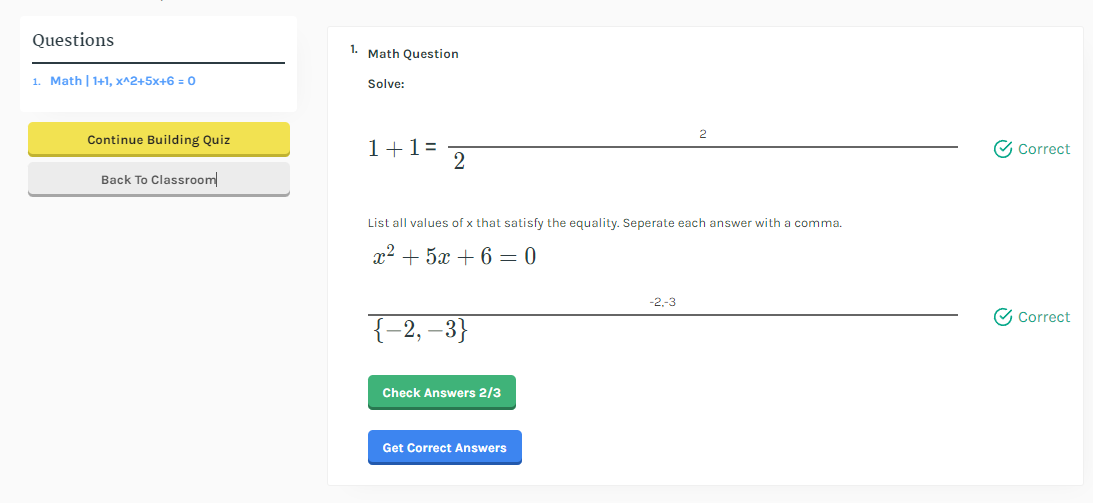1. When you have a random variable in your question, refreshing the page will cause its value to change.

*Conclusion

And that’s it! That’s how you create and automatically randomize your math questions in the Arlinear Quiz Builder. Feel free to drop a comment with a question and we’ll happily answer it for you :)

*You must be logged in to comment or like posts*